## 31-ed2 / 31-edo / 31-ET / 31-tone equal-temperament

[Joe Monzo]

#### 31-tone equal-temperament in standard meantone notation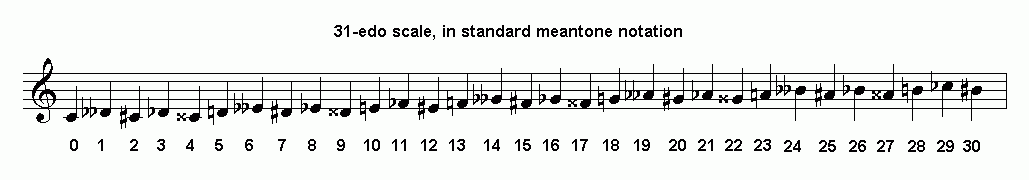Below is a tabulation of approximate and exact cents values for 31-edo, arranged in descending scalar order:

```                 31-edo
-- note name --  degree     ~cents      exact cents
C ............... 31     1200          1200
B# .. 30     1161.290323   1161 + 09/31
Cb .............. 29     1122.580645   1122 + 18/31
B ... 28     1083.870968   1083 + 27/31
Ax .... 27     1045.16129    1045 + 05/31
Bb .. 26     1006.451613   1006 + 14/31
A# .... 25      967.7419355   967 + 23/31
Bbb . 24      929.0322581   929 + 01/31
A ..... 23      890.3225806   890 + 10/31
Gx ...... 22      851.6129032   851 + 19/31
Ab .... 21      812.9032258   812 + 28/31
G# ...... 20      774.1935484   774 + 06/31
Abb ... 19      735.483871    735 + 15/31
G ....... 18      696.7741935   696 + 24/31
Fx ........ 17      658.0645161   658 + 02/31
Gb ...... 16      619.3548387   619 + 11/31
F# ........ 15      580.6451613   580 + 20/31
Gbb ..... 14      541.9354839   541 + 29/31
F ......... 13      503.2258065   503 + 07/31
E# .......... 12      464.516129    464 + 16/31
Fb ........ 11      425.8064516   425 + 25/31
E ........... 10      387.0967742   387 + 03/31
Dx ............  9      348.3870968   348 + 12/31
Eb ..........  8      309.6774194   309 + 21/31
D# ............  7      270.9677419   270 + 30/31
Ebb .........  6      232.2580645   232 + 08/31
D .............  5      193.5483871   193 + 17/31
Cx ..............  4      154.8387097   154 + 26/31
Db ............  3      116.1290323   116 + 04/31
C# ..............  2       77.41935484   77 + 13/31
Dbb ...........  1       38.70967742   38 + 22/31
C ...............  0        0             0
```

Each equal-temperament belongs to several different temperament families, based on the particular promos which it tempers out. 31-edo is historically one of the most important, primarily because it is a member of the meantone family, and in particular because it closely resembles 1/4-comma meantone. Note that because 31-edo's cardinality is a prime-number, any of 31-edo's intervals may be used as a generator to generate the entire tuning.

. . . . . . . . .
###### 31-edo as approximation of 1/4-comma meantone

Meantone temperaments are classified as "linear temperaments", and belong to the category of linear temperaments called "chain of 5ths" tunings, because they are analagous to the long-traditional pythagorean tuning, in that they use a generator which is a type of "perfect-5th", albeit one which is tempered so that its interval size is slightly smaller than the "pure" pythagorean "5th" (the pythagorean "5th" is ~702 cents). Using "C" as the name for the reference pitch, any "chain of 5ths" tuning (i.e., any tuning which uses a generator which is that tuning's version of the "perfect-5th") may be schematically illustrated as follows:

```note names:           Bb   F   C   G   D   A   E   B  F#
... --+---+---+---+---+---+---+---+---+-- ...
generator-5ths:       -2  -1   0  +1  +2  +3  +4  +5  +6
```

As can be seen from the tabulation of 31-edo cents values above, the closest approximation to the pythagorean "5th" in 31-edo is given by 18 degrees of 31-edo, an interval with a ratio of ~1.495517882 and a logarithmic size of 696 + 24/31 (~696.7741935) cents.

All meantone tunings contain subsets which are diatonic scales consisting of two different "step" sizes, a "Large" and a "small", which may be designated L and s. In all meantone tunings, the large interval (L) is the "whole-step" or "whole-tone", the interval of a "major 2nd", and is found by traversing +2 generator "5ths" and reducing by 1 octave; thus, in 31-edo, the L is:

```C = n0

first, stack 2 generator "5ths":

8ve  gen         ~ratio               cents      31-edo degrees
2^(((18*gen)/31)+8ve)

G   [ 0  +1]        1.495517882         696 + 24/31       18
+ 5th [ 0  +1]      * 1.495517882      +  696 + 24/31     + 18
--------------      -------------      --------------     ----
D   [ 0  +2]        2.236573736        1393 + 17/31       36    =  2 generator "5ths" (= major-9th)

then, reduce by 1 octave to normalize:

8ve  gen         ~ratio               cents      31-edo degrees
2(((18*gen)/31)+8ve)

D   [ 0  +2]        2.236573736        1393 + 17/31       36
- 8ve [ 1   0]      ÷ 2                - 1200             - 31
--------------      -------------      --------------     ----
D   [-1  +2]        1.118286868         193 + 17/31        5    =  major-2nd = L
```

Here is another example, which uses notes derived from +1 and -1 generators, where the -1 generator has already been octave normalized:

```C = n0
8ve  gen         ~ratio               cents      31-edo degrees
2(((18*gen)/31)+8ve)                    ((18*gen) mod 31)

G  [ 0  +1]        1.495517882         696 + 24/31        18
- F  [+1  -1]     ÷  1.337329378       - 503 + 07/31      - 13
-------------      -------------      ---------------     ----
*  [-1  +2]        1.118286868         193 + 17/31         5

* This always results in a change of letter-name.
```

This is the "mean-tone" which occurs almost exactly midway between the two just-intonation "whole-tones" with ratios of 10:9 and 9:8. (The exact mean-tone is that of 1/4-comma meantone.)

By continuing the process of stacking generators and normalizing, one may obtain the diatonic major scale. As an example, on the linear lattice-diagram illustrated above, the C-major scale appears as a subset of the lattice, between generators -1 and +5 inclusive. Regardless of the actual pitches (frequencies, or ratios, or cents values, etc.), the major scale whose tonic is the reference pitch (in this case, C) maps this way in any chain-of-5ths tuning.

In the table below, the "8ve" (octaves) and "gen" (generator 5ths) columns on the left show the generic [period, generator] mapping of pitches in the C-major scale for all members of the meantone family, and the "~ratio", "~cents", and "31-edo degrees" columns show the values associated with the mapping of the C-major scale specific to 31-edo:

```C = n0
8ve  gen         ~ratio               cents      31-edo degrees
2(((18*gen)/31)+8ve)                    ((18*gen) mod 31)

B  [-2   5]        1.870243098        1083 + 27/31       28
A  [-1   3]        1.672418009         890 + 10/31       23
G  [ 0   1]        1.495517882         696 + 24/31       18
F  [ 1  -1]        1.337329378         503 + 07/31       13
E  [-2   4]        1.25056552          387 + 03/31       10
D  [-1   2]        1.118286868         193 + 17/31        5
C  [ 0   0]        1                     0                0

(compare the "gen" values with those in the "chain of 5ths" linear lattice-diagram above)
```

In all meantone tunings, the small interval (s) is the "half-step", which is also the diatonic-semitone and the interval of a "minor 2nd", and is found by traversing -5 generator "5ths" (which is equivalent to +5 generator "4ths"). As can be seen in the "chain of 5ths" schematic above, this may be found twice in any major scale: between the octave above the tonic (reference note) and the leading-tone or major-7th, and also between the perfect-4th and the major-3rd. In 31-edo, the s is thus:

```C = n0

s between octave and major-7th:

8ve  gen         ~ratio               cents      31-edo degrees
2(((18*gen)/31)+8ve)

C  [+1   0]        2.                  1200               31
- B  [-2  +5]     ÷  1.870243098       - 1083 + 27/31     - 28
-------------      -------------      ---------------     ----
*  [+3  -5]        1.069379699          116 + 04/31        3

s between perfect-4th and major-3rd:

8ve  gen         ~ratio               cents      31-edo degrees
2(((18*gen)/31)+8ve)                    ((18*gen) mod 31)

F  [+1  -1]        1.337329378         503 + 07/31        13
- E  [-2  +4]     ÷  1.25056552        - 387 + 03/31      - 10
-------------      -------------      ---------------     ----
*  [+3  -5]        1.069379699         116 + 04/31         3

* This always results in a change of letter-name.
```

In both historical chronology and the method of scale construction by generators, the chromatic-semitone first emerges in meantone as the interval between B and Bb:

```C = n0
8ve  gen          ~ratio               cents      31-edo degrees
2(((18*gen)/31)+8ve)                    ((18*gen) mod 31)

Bb [+2  -2]        1.788449866        1006 + 14/31        26
- B  [-2  +5]      ÷ 1.870243098      - 1083 + 27/31      - 28
-------------      -------------      ---------------     ----
b [+4  -7]        0.956265989         -77 + 13/31        29 = -2 mod 31
```

The "round-b" or "soft-b" was used to notate Bb, and eventually evolved into the "flat" symbol, defined as above. This signifies a flattening (lowering in pitch) by 2 degrees of 31-edo.

B-natural was notated as "square-b" or "hard-b". Gradually, as the scale was extended, this "square-b" evolved into both the "natural" and "sharp" symbols, and also resulted in the Eastern European practice of calling "B-flat" simply "b", with "B-natural" called "h".

```C = n0
8ve  gen         ~ratio               cents      31-edo degrees
2(((18*gen)/31)+8ve)                    ((18*gen) mod 31)

F# [-3  +6]        1.398490998         580 + 20/13         15
- F  [+1  -1]     ÷  1.337329378       - 503 + 07/31       - 13
-------------      -------------      ---------------      ----
# [-4  +7]        1.045734148         +77 + 13/31          2
```

This is the interval of the "augmented unison" or "augmented prime", also known as the "chromatic semitone", and signifies a sharpening (raising in pitch) by 2 degrees of 31-edo.

Extending the system still further, to Eb -3 on the flat side and to D# +9 on the sharp side, we find a smaller interval, the "diminished 2nd":

```C = n0
8ve  gen         ~ratio               cents      31-edo degrees
2(((18*gen)/31)+8ve)                    ((18*gen) mod 31)

Eb [+2  -3]        1.195873274         309 + 21/31          8
- D# [-5  +9]     ÷  1.169430766       - 270 + 30/31        - 7
-------------      -------------      ---------------      ----
[+7 -12]        1.022611436          38 + 22/31          1
```

Extending the system still further, to Cb -7 on the flat side and to B# +12 on the sharp side, we find a new interval, the "augmented 7th" minus an "8ve", which, in 31-edo, is exactly the same size as the "diminished 2nd" discovered above:

```C = n0
8ve   gen         ~ratio                cents      31-edo degrees
2(((18*gen)/31)+8ve)                    ((18*gen) mod 31)

B# [ -6 +12]        1.95577707         1161 + 09/31         30
- Cb [ +5  -7]     ÷  1.912531979      - 1122 + 18/31      - 29
--------------      -------------     ----------------     ----
[-11 +19]        1.022611436          38 + 22/31         1
```

Finally, extending the system to Cbb -14 on the flat side and Ax +17 on the sharp side, we find the point where the system closes:

```C = n0
8ve   gen         ~ratio               cents      31-edo degrees
2(((18*gen)/31)+8ve)                    ((18*gen) mod 31)

Cbb [ +9 -14]        1.828889285        1045 + 05/31       27
- Ax  [ -9 +17]     ÷  1.828889285      - 1045 + 05/31     - 27
---------------      -------------      ------------       ----
[+18 -31]        1                     0                0
```

Below is a diagram of the 31-edo "circle-of-5ths" in this typical meantone usage, giving the 31-edo degree-numbers in the cubes and the letter-name notation alongside, showing the closure of the system between Gbb -13 = Ex +18 generators and Cbb -14 = Ax +17 generators: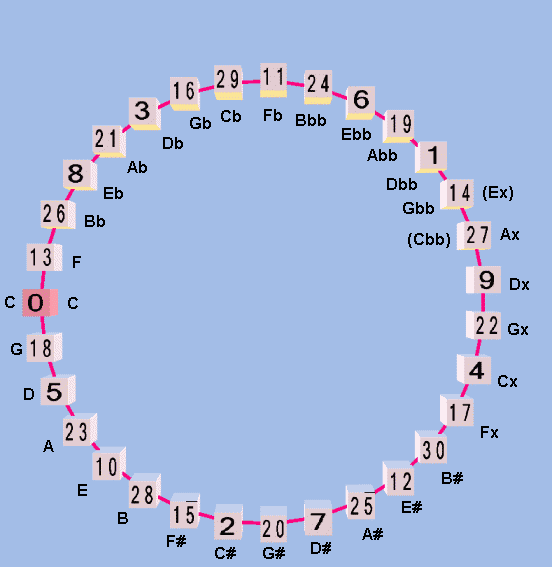Below is a pitch-height graph of the complete 31-edo circle-of-5ths, shown as a meantone chain, centered on C. The red arrows connect the enharmonically equivalent notes at each end of the chain which are exactly the same pitch.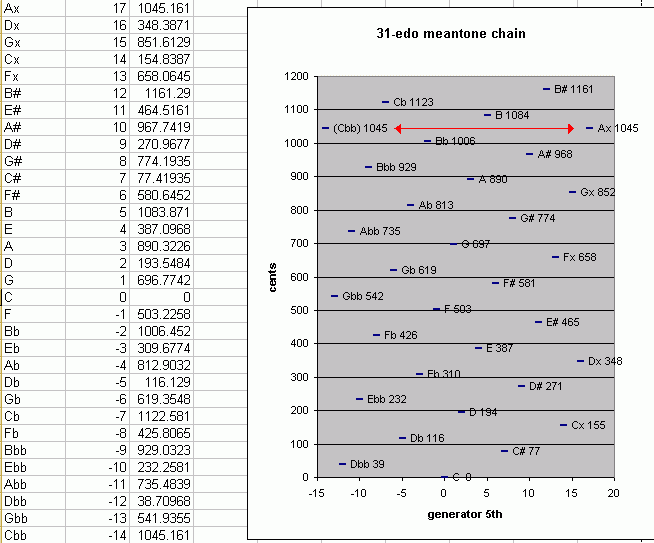Below is the same circle-of-5ths-as-meantone-chain, shown on the regular 5-line staff notation, with a slur connecting the enharmonically equivalent notes.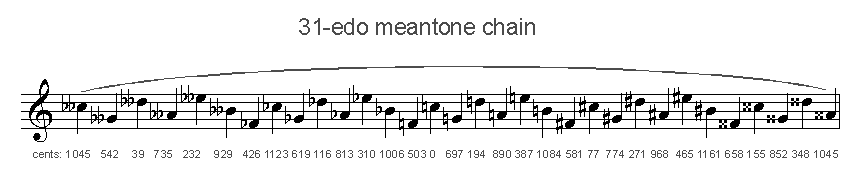Below is a circular diagram of 31-edo with letter notation of its typical meantone usage, showing the degrees of 31-edo arranged according to pitch-height scalar order around the circle which represents the "8ve".

#### 31-et circle of scale degrees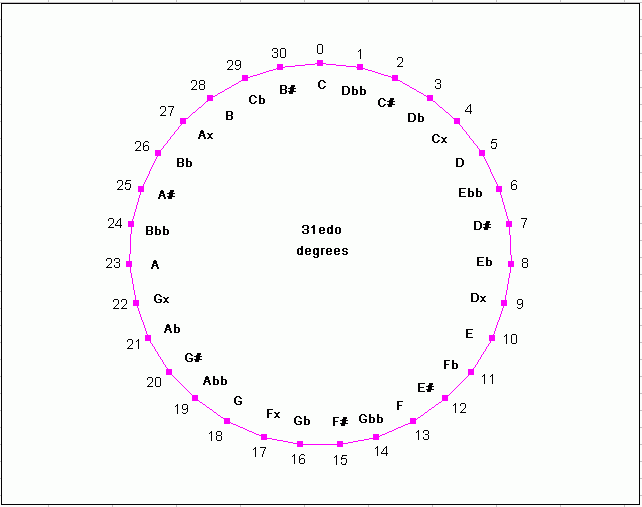When used as a meantone, 31-edo is also intended to approximate ratios containing prime-factor 5 -- thus, a lattice of the just-intonation pitches being approximated becomes planar (2-dimensional) rather than simply linear (1-dimensional), i.e., another dimension is added to represent prime-factor 5. Below is a 2-dimensional 5-limit bingo-card lattice-diagram, showing a typical periodicity tiling of the 31-edo representations of 5-limit ratios, with a typical spelling in a chain from Gbb at -13 generators to Ax at +17 generators where C=n0 -- it would be difficult to find music from the "common-practice" (c. 1600-1900) repertoire which has notes falling outside this range.

Exponents of 3 run across the top row and exponents of 5 run in a column down the left side; C n0 is outlined in heavy black; syntonic-comma equivalents (which have the same spelling) are in a light shade of grey; enharmonically-equivalent pitches (which have a different spelling but are the same pitch as those in the central block) are in a darker shade of grey. Integers designate the degrees of 31-edo.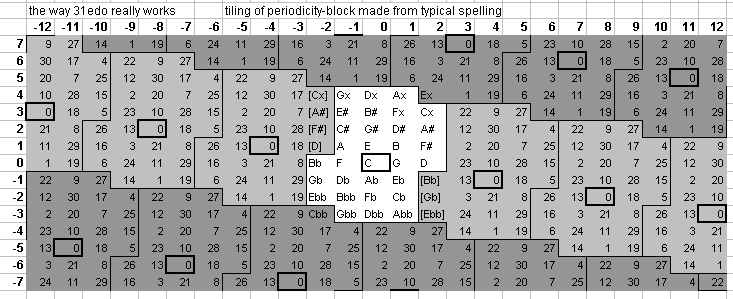When used as a meantone, 31-edo provides not only a circle of "5ths" (i.e., tempered approximation of the 3:2 ratio), but also a circle of "major-3rds" (i.e., tempered approximation of the 5:4 ratio) -- in our example C-based lattice, this circle can be spelled C-E-G#-B#-Fbb-Abb-Cb-Eb-G-B-D#-Fx-Cbb-Ebb-Gb-Bb-D-F#-A#-Cx-Gbb-Bbb-Db-F-A-C#-E#-Gx-Dbb-Fb-Ab-C. The closed geometry which represents both of these circles simultaneously takes the form of a torus, with both circles twisted to form two interconnected spirals which outline the surface of the torus. Below is a graphic adapted from a Musica lattice (based on A instead of C), showing 31-edo as a torus, with the circle of 5ths as a closed tight spiral colored magenta, and the other closed spiral, representing major-3rds, wider and colored green.

#### 31-edo 3,5-primespace toroidal lattice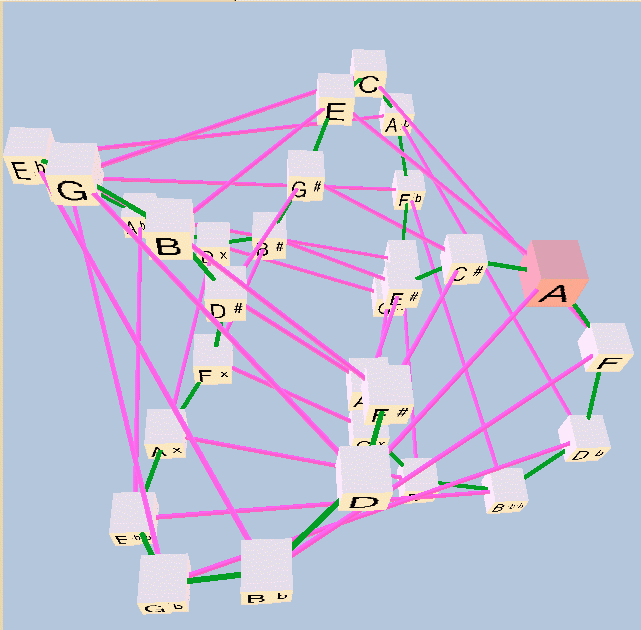(Be aware that 31-edo also belongs to other temperament families! ... namely, miracle, würschmidt, and orwell.)

. . . . . . . . .

Below is a table showing how some of the most common 11-limit just-intonation ratios, and some others up to the 41-limit, are mapped to 31-edo, using the closest-fit mappings:

```
====================================
edomap.py (c)2008-12-23 by Joe Monzo
====================================

Finds the EDO mappings for a set of ratios

=============== new run =====================

31 -edo floating-point mappings:

prime edo-steps   step-error  edo-map
2 =  31.000000    +0.00 -->     31
3 =  49.133838    -0.13 -->     49
5 =  71.979771    +0.02 -->     72
7 =  87.028003    -0.03 -->     87
11 = 107.242380    -0.24 -->    107
13 = 114.713631    +0.29 -->    115
17 = 126.711348    +0.29 -->    127
19 = 131.685753    +0.31 -->    132
23 = 140.230421    -0.23 -->    140
29 = 150.597411    +0.40 -->    151
31 = 153.580086    +0.42 -->    154
37 = 161.493054    -0.49 -->    161
41 = 166.084112    -0.08 -->    166

integer (i.e., true) mappings, compared with cents-value of actual prime

map  2 -->     31 = 1200.000000 cents <-- 1200.000000  + 0.0 cents
map  3 -->     49 = 1896.774194 cents <-- 1901.955001  - 5.2 cents
map  5 -->     72 = 2787.096774 cents <-- 2786.313714  + 0.8 cents
map  7 -->     87 = 3367.741935 cents <-- 3368.825906  - 1.1 cents
map 11 -->    107 = 4141.935484 cents <-- 4151.317942  - 9.4 cents
map 13 -->    115 = 4451.612903 cents <-- 4440.527662  +11.1 cents
map 17 -->    127 = 4916.129032 cents <-- 4904.955410  +11.2 cents
map 19 -->    132 = 5109.677419 cents <-- 5097.513016  +12.2 cents
map 23 -->    140 = 5419.354839 cents <-- 5428.274347  - 8.9 cents
map 29 -->    151 = 5845.161290 cents <-- 5829.577194  +15.6 cents
map 31 -->    154 = 5961.290323 cents <-- 5945.035572  +16.3 cents
map 37 -->    161 = 6232.258065 cents <-- 6251.344039  -19.1 cents
map 41 -->    166 = 6425.806452 cents <-- 6429.062406  - 3.3 cents

--------------

examples:

ratio      -->     31 -edo mapping:

ratio      cents   error      edo    cents    name

2:1     = 1200.0  + 0.0 -->  31/31 = 1200.0  (octave)

31:16    = 1145.0  +16.3 -->  30/31 = 1161.3  (31st harmonic)

4096:2187  = 1086.3  +36.3 -->  29/31 = 1122.6  (pythagorean diminished-8ve)

243:128   = 1109.8  -25.9 -->  28/31 = 1083.9  (pythagorean major-7th)
15:8     = 1088.3  - 4.4 -->  28/31 = 1083.9  (just major-7th, 5*3)
13:7     = 1071.7  +12.2 -->  28/31 = 1083.9  (tridecimal superminor-7th)

11:6     = 1049.4  - 4.2 -->  27/31 = 1045.2  (undecimal submajor-7th)
20:11    = 1035.0  +10.2 -->  27/31 = 1045.2  (undecimal superminor-7th)
29:16    = 1029.6  +15.6 -->  27/31 = 1045.2  (29th harmonic)

9:5     = 1017.6  -11.1 -->  26/31 = 1006.5  (just minor-7th)
16:9     =  996.1  +10.4 -->  26/31 = 1006.5  (pythagorean minor-7th)

7:4     =  968.8  - 1.1 -->  25/31 =  967.7  (7th harmonic)

12:7     =  933.1  - 4.1 -->  24/31 =  929.0  (septimal supermajor-6th)

27:16    =  905.9  -15.5 -->  23/31 =  890.3  (pythagorean major-6th)
5:3     =  884.4  + 6.0 -->  23/31 =  890.3  (just major-6th)

18:11    =  852.6  - 1.0 -->  22/31 =  851.6  (undecimal neutral-6th)
13:8     =  840.5  +11.1 -->  22/31 =  851.6  (13th harmonic)

8:5     =  813.7  - 0.8 -->  21/31 =  812.9  (just minor-6th)
128:81    =  792.2  +20.7 -->  21/31 =  812.9  (pythagorean minor-6th)

6561:4096  =  815.6  -41.4 -->  20/31 =  774.2  (pythagorean augmented-5th)
11:7     =  782.5  - 8.3 -->  20/31 =  774.2  (undecimal augmented-5th)
25:16    =  772.6  + 1.6 -->  20/31 =  774.2  (just augmented-5th)
14:9     =  764.9  + 9.3 -->  20/31 =  774.2  (septimal subminor-9th)

3:2     =  702.0  - 5.2 -->  18/31 =  696.8  (perfect-5th)

16:11    =  648.7  + 9.4 -->  17/31 =  658.1  (11th subharmonic)

23:16    =  628.3  - 8.9 -->  16/31 =  619.4  (23rd harmonic)
10:7     =  617.5  + 1.9 -->  16/31 =  619.4  (septimal large-tritone)
1024:729   =  588.3  +31.1 -->  16/31 =  619.4  (pythagorean diminished-5th)

729:512   =  611.7  -31.1 -->  15/31 =  580.6  (pythagorean augmented-4th)
7:5     =  582.5  - 1.9 -->  15/31 =  580.6  (septimal small-tritone)

11:8     =  551.3  - 9.4 -->  14/31 =  541.9  (11th harmonic)

4:3     =  498.0  + 5.2 -->  13/31 =  503.2  (perfect-4th)

21:16    =  470.8  - 6.3 -->  12/31 =  464.5  (septimal-4th, 7*3)

9:7     =  435.1  - 9.3 -->  11/31 =  425.8  (septimal supermajor-3rd)
41:32    =  429.1  - 3.3 -->  11/31 =  425.8  (41st harmonic)
14:11    =  417.5  + 8.3 -->  11/31 =  425.8  (undecimal diminished-4th)
8192:6561  =  384.4  +41.4 -->  11/31 =  425.8  (pythagorean diminished-4th)

81:64    =  407.8  -20.7 -->  10/31 =  387.1  (pythagorean major-3rd)
5:4     =  386.3  + 0.8 -->  10/31 =  387.1  (just major-3rd)

11:9     =  347.4  + 1.0 -->   9/31 =  348.4  (undecimal neutral-3rd)
39:32    =  342.5  + 5.9 -->   9/31 =  348.4  (39th harmonic, 13*3)

6:5     =  315.6  - 6.0 -->   8/31 =  309.7  (just minor-3rd)
19:16    =  297.5  +12.2 -->   8/31 =  309.7  (19th harmonic)
32:27    =  294.1  +15.5 -->   8/31 =  309.7  (pythagorean minor-3rd)
13:11    =  289.2  +20.5 -->   8/31 =  309.7  (tridecimal diminished-3rd)

19683:16384 =  317.6  -46.6 -->   7/31 =  271.0  (pythagorean augmented-2nd)
7:6     =  266.9  + 4.1 -->   7/31 =  271.0  (septimal subminor-7th)

37:32    =  251.3  -19.1 -->   6/31 =  232.3  (37th harmonic)
8:7     =  231.2  + 1.1 -->   6/31 =  232.3  (septimal tone)

9:8     =  203.9  -10.4 -->   5/31 =  193.5  (pythagorean major-2nd/tone)
10:9     =  182.4  +11.1 -->   5/31 =  193.5  (just minor-tone)

11:10    =  165.0  -10.2 -->   4/31 =  154.8  (undecimal small-tone/submajor-2nd)
35:32    =  155.1  - 0.3 -->   4/31 =  154.8  (35th harmonic, 7*5)
12:11    =  150.6  + 4.2 -->   4/31 =  154.8  (undecimal large-semitone)
13:12    =  138.6  +16.3 -->   4/31 =  154.8  (tridecimal minor-2nd)

14:13    =  128.3  -12.2 -->   3/31 =  116.1  (tridecimal major-2nd)
15:14    =  119.4  - 3.3 -->   3/31 =  116.1  (septimal chromatic-semitone)
16:15    =  111.7  + 4.4 -->   3/31 =  116.1  (just diatonic-semitone)
17:16    =  105.0  +11.2 -->   3/31 =  116.1  (septendecimal semitone)
256:243   =   90.2  +25.9 -->   3/31 =  116.1  (pythagorean minor-2nd/limma)

2187:2048  =  113.7  -36.3 -->   2/31 =   77.4  (pythagorean augmented-prime/apotome)
25:24    =   70.7  + 6.7 -->   2/31 =   77.4  (just chromatic-semitone)

33:32    =   53.3  -14.6 -->   1/31 =   38.7  (33rd harmonic, 11*3)

1:1     =    0.0  + 0.0 -->   0/31 =    0.0  (prime)

some commas:

3-limit:
531441:524288 =   23.5  -62.2 -->  -1/31 =  -38.7  (pythagorean-comma)

5-limit:
648:625       =   62.6  -23.9 -->   1/31 =   38.7  (major-diesis)
250:243       =   49.2  +28.3 -->   2/31 =   77.4  (maximal-diesis)
128:125       =   41.1  - 2.3 -->   1/31 =   38.7  (enharmonic-diesis)
34171875:33554432 =   31.6  -31.6 -->   0/31 =    0.0  (ampersand-comma)
3125:3072      =   29.6  + 9.1 -->   1/31 =   38.7  (magic-comma)
81:80        =   21.5  -21.5 -->   0/31 =    0.0  (syntonic-comma)
2048:2025      =   19.6  +19.2 -->   1/31 =   38.7  (diaschisma)
2109375:2097152   =   10.1  -10.1 -->   0/31 =    0.0  (semicomma)
15625:15552     =    8.1  +30.6 -->   1/31 =   38.7  (kleisma)
32805:32768     =    2.0  -40.7 -->  -1/31 =  -38.7  (skhisma)

7-limit:
36:35        =   48.8  -10.1 -->   1/31 =   38.7  (septimal-diesis)
49:48        =   35.7  + 3.0 -->   1/31 =   38.7  (septimal-comma-3 - 7/6 : 8/7)
50:49        =   35.0  + 3.7 -->   1/31 =   38.7  (septimal-comma-2)
64:63        =   27.3  +11.4 -->   1/31 =   38.7  (septimal-comma)
225:224       =    7.7  - 7.7 -->   0/31 =    0.0  (septimal-kleisma)

11-limit:
22:21        =   80.5  - 3.1 -->   2/31 =   77.4  ()
33:32        =   53.3  -14.6 -->   1/31 =   38.7  (undecimal-diesis)
45:44        =   38.9  - 0.2 -->   1/31 =   38.7  ()
55:54        =   31.8  + 6.9 -->   1/31 =   38.7  ()
56:55        =   31.2  + 7.5 -->   1/31 =   38.7  ()
121:120       =   14.4  -14.4 -->   0/31 =    0.0  (11/10 : 12/11 comma)
```

Below is a graph showing the degrees of 31-edo as the Y-axis grid, with the pitch of the 11-limit JI ratios plotted against it: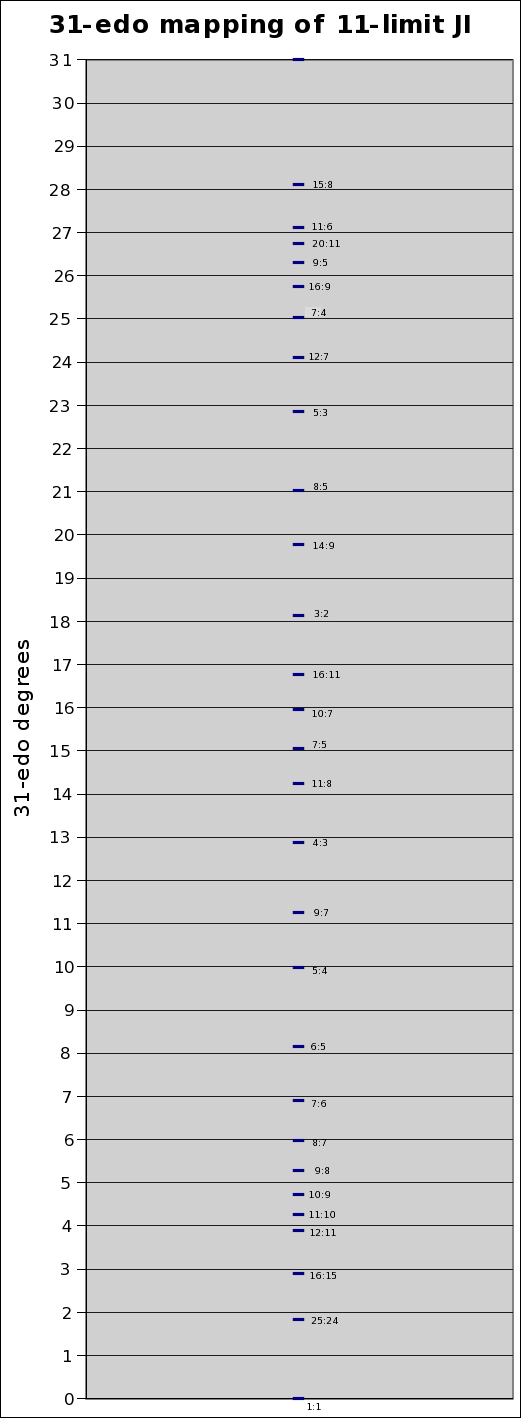Below is a graph showing the degrees of 31-edo along the X-axis, with the Y-value of zero representing the pitch of the 31-edo degrees, and the deviation of the JI ratios from the 31-edo degrees plotted against that: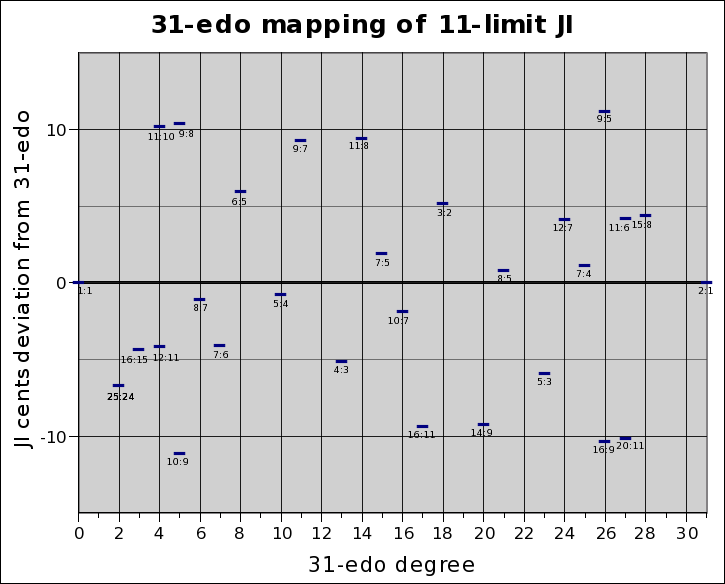. . . . . . . . .
 31=0 2:1 = 1:1 30 43:22 , 45:23 , 88:45 , 133:68 , 221:113 , 575:294 29 21:11 , 44:23 , 153:80 28 15:8 , 43:23 , 101:54 , 245:131 27 11:6 , 64:35 , 139:76 , 342:187 , 481:263 26 9:5 , 25:14 , 34:19 , 59:33 , 93:52 25 7:4 , 397:227 24 12:7 , 53:31 , 65:38, 118:69 , 183:107 , 301:176 23 5:3 , 97:58 22 18:11 , 157:96 21 8:5 , 435:272 , 443:277 20 11:7 , 25:16 , 61:39 , 208:133 19 26:17 18 3:2 , 166:111 17 19:13 , 117:80 , 253:173 , 370:253 16 10:7 , 123:86 , 133:93 15 7:5 , 186:133 14 11:8 , 15:11 , 26:19 , 67:49 , 93:68 , 253:185 13 4:3 , 111:83 12 17:13 11 9:7 , 23:18 , 55:43 , 78:61 , 133:104 10 5:4 9 11:9 , 192:157 8 6:5 , 55:46 , 116:97 7 7:6 , 69:59 , 283:242 , 352:301 6 8:7 , 223:195 , 231:202 5 9:8 , 19:17 , 85:76 , 104:93 4 11:10 , 12:11 , 35:32 , 152:139 , 187:171 3 15:14 , 31:29 , 77:72 , 185:173 , 262:245 2 22:21 , 23:22 , 160:153 1 42:41 , 43:42 , 44:43 , 45:44 , 181:177 0 1:1 progressing to the right = greater accuracy of approximation and greater complexity
. . . . . . . . .

The tonalsoft.com website is almost entirely the work of one person: me, Joe Monzo. Please reward me for my knowledge and effort by selecting the highest level of financial support that you can afford. Thank you.

 support level patron: \$50 USD savior: \$100 USD angel of tuning: \$500 USD microtonal god: \$1000 USD friend: \$25 USD donor: \$5 USD